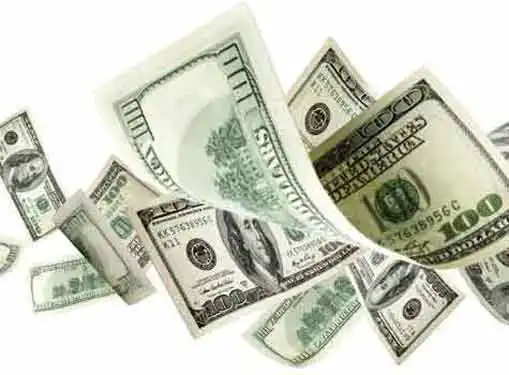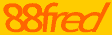# Understanding Discounted Cash Flow

Written by Bobby Jan for Gaebler Ventures

Discounted cash flow is a very important concept in business valuation. Discounted cash flow, or DCF, is an income approach to business valuation.

Using the concept of the time value of money, what discounted cash flow (DCF) does is determine the present value of a business by discounting all of its future cash flow.PV= (CFt)/ (1+d) ^t

What this equation says is that the present value (PV) of something after t periods is equal to the cash flow (CF) after t periods (hence the subscript "t") divided by the discount rate (1+d) per period to the t power.

For example, let us imagine that your friend Joe wants to sell you a contract. The contact says for X amount of dollars, he will give you \$10,000 three years later. To make things easier, let us assume that you are 100% sure that Joe will honor this contract since he is your good friend and have millions of dollars in the bank. You must, however, bid with another friend for this contact by offering a lump sum to be paid immediately to Joe. How much is the contract worth?

We can use the simple formula above to solve this problem. First, we know that the cash flow after three years is \$10,000--that is a given. Now, we must determine the discount rate. Let us assume that you could buy a three year CD and get 5% on your money every year.

Since CDs are insured by the government, there is almost no risk involved with this investment. If you didn't pay Joe X dollars for the contract, you could put it in the CD and get X *(1+0.05) ^3 after three years (this means your money will compound by 5% for three years). You can use now substitute 0.05 for "d" and get a discount rate of 1.05 per years. Since the payoff isn't until three years later, you can substitute the "t" with 3.

Now we have present value=\$10,000/(1.05)^3=\$8638.376. If all the previous assumptions are true, then the contract is worth no more than the present value as determined by the simple equation.

Now, let us expand this equation: PV= (CFt)/ (1+d) ^t + (CFt+1)/ (1+d) ^(t+1) + (CFt+2)/ (1+d) ^(t+2) +...

This more complication equation is essentially the same as the previous equation. The only difference is that we consider many periods of cash flow. This new equation better suits business valuation.

Let's consider a simple example: you want to buy a business on January 1st. By the end of the year, the business will make \$100,000 in profit. Profits will not grow but \$100,000 a year profit will continue forever with no risk. Let us continue using the 5% discount rate.

To solve this problem, we will again plug everything into the equation. We get:

PV= (\$100,000) /(1.05) + (\$100,000)/(1.05) ^2 +...= about \$2.1 million. You could easily do this on excel.

Of course, these examples are unrealistic but they are only the first steps to understanding the discounted cash flow approach to business valuation. There are many sophisticated methods for projecting the cash flow and determining the discount rate. More accurate projections of cash flow and discount rate will give better results.

Cheng Ming (Bobby) Jan is an Economics major at the University of Chicago who has a strong interest in entrepreneurship and investing.

## Conversation Board